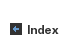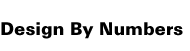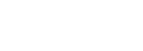DBN Vocabulary by Zach Lieberman and Rusty Soo-Tho paper the background color for the page. Default is white. Fills the page with color. Should come first, unless you want to clear the screen after you've drawn. Takes one parameter - a number between 0 and 100. 0 is white ( think 0% black) and 100 is black (100% black). Some examples : paper 0 paper 50 paper 100 pen used to set the pen color. Default is black. Takes one parameter - a number between 0 and 100. 0 is white ( think 0% black) and 100 is black (100% black). When you use the line or dot (set) command, it renders in that color. The color can be changed, as well. for example: pen 30 line 0 0 100 100 pen 60 line 0 100 100 0 pen 80 line 50 0 50 50 line takes 4 parameters : x1, y1, x2, y2. These are the x and y coordinates of both ends of the line. The order that the ends are typed in doesn't matter. for example: line 0 0 100 100 = line 100 100 0 0 and so on. There are some good examples of reasons why you won't see a line on page 33 -- if the line is the same color as the paper, if the line is outside of the 0, 0 to 100, 100 boundaries, or if the paper command is used after the line command. calculations are a way of asking the program to do math for us. For example, if we said: paper (50 - 10) the computer would take the "(" as a signal to "do something". In this case, we are saying do addition. It's important that parentheses are used, because the computer gets easily tripped up with: paper 50 - 10. It sees only paper 50 and then is confused about "- 10" It also is used to organize the order of operations. For example, specifying ((10 - 5) * 13) forces the interior "(" parenthesis to be evaluated first. Order of operations is a concept we'll get into a little later. comments a way of adding text to programs in way where they are skipped over as code is evaluated. They are often used to provide descriptions of the code. As well, they are used to break up blocks of code and increase legability. Most languages have the ability to contain comments. Often, the way you specify comments is different for each language. Java, javascript and actionscript use "//". Java uses "/*" to open multi-line comments and "*/" to close. Perl uses "#". parameters these are numerical arguments that commands take. For example, paper takes one parameter (the color of the paper). The order in which you pass the parameters is very important. For example, 10 paper won't work. Also paper10 will fail. DBN wants parameters to come after the command. A variable can be used as a parameter. We will cover this later. Some parameter examples : paper 10 // 1 parameter "10" pen 40 // 1 parameter "40" line 20 20 50 10 // 4 parameters The separator in DBN is a space. Other languages have other ways of passing and separating commands. Javascript uses parentheses and commas i.e. makeMove(3, 4) . coordinates different programming languages have different ways of referring to their coordinate system. The most important is the (0,0) point, or the origin. in DBN, the origin is located at the bottom left corner. The top right corner is (100,100). The box is 101 pixels by 101 pixels. In contrast, java refers to the top left corner as the origin. The DBN interface has a little ruler attached for your reference. variable A variable is basically a place holder for numeric information. You choose a name for your variable (ex: myHeight) and assign it a value that you want it to keep track of (ex: 60). In DBN, this would look like: Set myHeight 60 You can change the value of the variable later on (ex: as your height grows). Think of a variable as a container that you are asking to hold something for you, like your jar of blue gumballs (mmm... Gumballs....). And you can change the value of that variable (hence the name "variable") as easily as if you took another gumball out of the jar. Why are variables significant? a) They allow us to create relationships between different parts of our program. For example, let's say you wanted the color of a rectangle to change depending on its position on the screen. Those two values  position and color  have a relationship, and by setting a variable, your program can monitor that relationship for you. b) They allow us to abstract as we code. Instead of specifying a triangle at the specific points, we can program a general triangle which takes different values for its variables. Programmers are by nature lazy (see Programming Perl for an explanation of why that is) and by using variables, we can write more powerful, abstracted code. set This is the command that DBN requires before you assign a value to a variable. The syntax looks like this: Set myHeight 60 myHeight is the name of the variable. 60 is the value that you are putting in that variable. The Set command is also used for dots (drawing on individual pixels). The syntax in that situation looks like this: Set [50 50] 100 This puts a dot (which has the Pen color) in the middle of the page. [50 50] is the x and y position of the dot and 100 is the color. naming Having a consistent naming scheme is very important, especially when you program get larger and larger. There's really no reason to give them super technical names like dt145 or x4_id9 when my_width or big_red_dot will work just as well and will be easier to remember. One letter names, although used often in DBN, can be quite confusing at times. Your best bet is to use logical, concise names that will make your code legible for others and yourself. There are some limitations to what you can name your variables. You can't use any words that are already used as commands (set, paper, pen...) and you can't start a variable name with a number. Other than that, you can use any style you want. For multiple word variables, it's common to either separate the words with an _underscore (big_red_dot) or by capitalizing each word, starting with the second (bigRedDot). copy Making one variable equal to another is called copying. Basically you are copying the contents of one into the other. Here's the example from the book: Paper 0 Set G 25 Pen G // we create a variable H which has the value of G... Set H G Line H 0 H 100 Saying "Set H G" gives H the value of G (in this case 25). In this program, by linking H and G, the position of the vertical line is determined by its color. Try this in DBN and change G in the Set G line. repeat A repeat loop is a way of the telling the computer to do something more than one time. The format is like this : Repeat variableName start end {   code to be executed } Basically, a repeat loop takes a starting value as a parameter, an end value, then counts its way up (or down), each time through setting the value of the variable to whatever it's counted to. It's one of those things that makes a lot more sense when you see it in action. a practical example : Repeat count 25 50 {   line 0 count 100 count } nested repeat Whenever DBN sees a repeat loop, it will do it till it's done. Repeat loops can be nested in repeat loops. Here's an example : Repeat a 0 10 {   Repeat b 0 10   {     Set [a b] (a*b)   } } The important concept here, is that for every one of the outer loops (a = 0, a = 1, a = 2) we do the eleven inner loops : (b = 0, b = 1, b = 2...). Give it a try and it'll make sense. same? Checks whether two parameters passed to it are the same. If so,it executes whatever is in the block directly below it. Same? a b {   // code to execute } parameters a and b can be variables or numbers (remember that variables in the end become numbers when DBN looks them up in memory) Same? 2 b {   // .... } checks if 2 and the value of "b" are equal. same? a width { } checks if the variable "a" and the variable "width" are equal. Same? 10 10 {   // .. } checks if 10 = 10, in case you were unsure of that ... notsame? Similar to same, except this checks for not equal ( in other circles commonly referred to as != with the ! meaning not). NotSame? a b {   // code to execute } parameters a and b can be variables or numbers (remember that variables in the end become numbers when DBN looks them up in memory) NotSame? 59 yo {   // .... } checks if 59 and the value of "yo" are not equal. NotSame? height mouseX { } checks if the variable "height" and the variable "mouseX" are equal. NotSame? 20 10 {   // .. } checks if 20 != 10. Can't you sleep better knowing DBN can evaluate this? smaller? Checks whether the first of two parameters passed to it is smaller than the second. If so, it executes whatever is in the block directly below it. (in other languages, the "less than" symbol (<) is used) Set a 25 Smaller? a 26 {   // code to execute } parameters a and b can be variables or numbers (remember that variables in the end become numbers when DBN looks them up in memory) Repeat a 0 10 {   Smaller? a 5   {     //draw a square   } } This code will draw 5 squares because the square code will only execute when "a" is 0, 1, 2, 3, and 4. notsmaller? This is the opposite of smaller. It evaluates if the first of the two parameters it is passed is greater than or equal to the second. If it is, all the code in the block below it will be executed. Set a 25 NotSmaller? a 24 {   // code to execute } parameters a and b can be variables or numbers (remember that variables in the end become numbers when DBN looks them up in memory) Repeat a 0 10 {   NotSmaller? a 5   {     //draw a square   } } This code will draw 6 squares because the square code will only execute when "a" is 5, 6, 7, 8, 9 and 10. command So far we have used the standard commands that come with dbn, like line, paper, pen etc. We can also create our own custom commands. A command is a block of code that we give a name and also specify how many parameters it needs to be passed. Command drawRect x1 y1 x2 y2 color {   Pen color   Line x1 y1 x1 y2   Line x1 y1 x2 y1   Line x2 y1 x2 y2   Line x2 y2 x1 y2 } First, we type the command "command" (confusing, eh?) to tell dbn that we are giving the block of code below a name (aka: a place in memory). After that we've typed five variables (x1 y1 x2 y2 color) to hold the values of the five parameters we require for our command to work (in this case it's the lower right corner coordinates, the upper left corner coordinates, and the color of the lines making the rectangle). The block of code that follows is what is going to be executed whenever we use that command. To call the command, we simply type into our program: drawRect 10 10 90 90 100 DBN sees the command and automatically plugs the values into the parameters in the order they were declared (x1=10, y1=10, x2=90.....) and then executes the code with those values. **It is important to define you commands BEFORE you ask DBN to execute them. Remember, the code is read line by line, starting from the top. If your code looks like this: drawRect 10 10 90 90 100 Command drawRect x1 y1 x2 y2 color {   Pen color   Line x1 y1 x1 y2   Line x1 y1 x2 y1   Line x2 y1 x2 y2   Line x2 y2 x1 y2 } DBN will hit the "drawRect..." line first and go "huh?" because you haven't yet taught it what drawRect means. We can also use the commands we have created to create other commands. This is nesting commands. Command pinwheel x y size color {   drawRect (x-size) y x (y+(size/2)) color   drawRect x y (x+(size/2)) (y+size) color   drawRect x (y-(size/2)) (x+size) y color   drawRect (x-(size/2)) (y-size) x y color } pinwheel 50 50 12 100 This creates a command called "pinwheel" that uses the previous drawRect command to place four squares in the shape of a pinwheel. All we have to do is give it the x & y of where we want it to go, the size of the wing, and the color we want it.